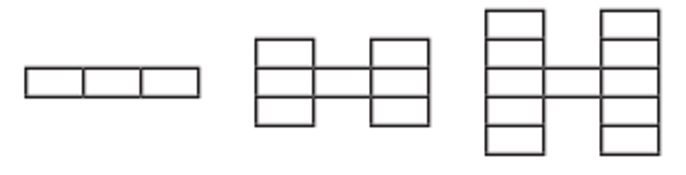Mathematics
Easy

Question

# Smith creates a pattern with rectangles.If the pattern continues, the number of rectangles required to make the 4th shape is ___.

## 15    19    20    25Hint:

## The correct answer is: 15

### The number of shape and number of triangles used to make the shape are as follows:First shape has 3 rectangles.Second shape has (3 + 4) = 7Third shape has (7 + 4) = 11If we see, the number of rectangles is increasing by 4. Smith started with 3 rectangles and with increasing number of shape, increased the number by 4.So, fourth shape will have (11 + 4) = 15 rectangles.The fourth shape will have 15 rectangles.

While solving questions, we should be careful about the sequence of the shapes.

### Related Questions to study#### With Turito Foundation.#### Get an Expert Advice From Turito.